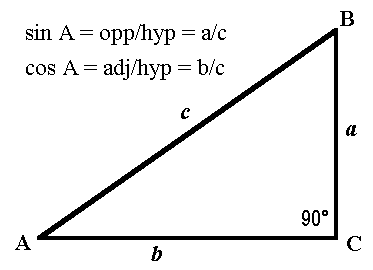# Right Triangle Angle And Side Calculator

This calculator requires the use of Javascript enabled and capable browsers.

This calculator is designed to give the two unknown factors in a right triangle, assuming two factors are known. This calculator is for a right triangle only! The factors are the lengths of the sides and one of the two angles, other than the right angle. All values should be in positive values but decimals are allowed and valid. Fill in two (only two) values then click on Calculate. The other two other modifiable values will be filled in, along with the angle 3 field. In a triangle, all interior angles total to 180 degrees. No two angles can total to 180 degrees or more. Angle C is always 90 degrees; angle 3 is either angle B or angle A, whichever is NOT entered. Angle 3 and Angle C fields are NOT user modifiable.

Again, this right triangle calculator works when you fill in 2 fields in the triangle angles, or the triangle sides. Angle C and angle 3 cannot be entered.

In case you need them, here are the Trig Triangle Formula Tables, the Triangle Angle Calculator is also available for angle only calculations.

 Unit Number Required Data EntrySide a Units Of Length Side b Units Of Length Side c Units Of Length Angle A or B Degrees Angle C Degrees Angle 3 Degrees Decimal Places 0 1 2 3 4 5 Maximum
Updated: 7/13/11

 Leave us a question or comment on FacebookSearch or Browse Our Site